## Archive for the ‘Code’ Category

Are you unsure if you need to include the space in your rules?

Most grammars will have this LEXER rule in their grammar:

```WS  :   ( ' '
| '\t'
| '\r'
| '\n'
) {\$channel=HIDDEN;}
;```

Now you’ll like a rule that needs to match “a sample string” (quotes included)? How will Antlr respond to the space?

or

You want to parse a command line like parameter alike string such as “operation /option1 /option2” (quotes not included).

```grammar test20091014;

//thanks to AntlrWorks 1.3 for it's useful grammar wizard.

prog	:	STRING+ OPTION*;

OPTION	:	'/' STRING;

STRING
:  '"' ( ESC_SEQ| ~('\\'|'"') )* '"'
|	ID
;

fragment ID  :	('a'..'z'|'A'..'Z'|'_') ('a'..'z'|'A'..'Z'|'0'..'9'|'_')*
;

WS  :   ( ' '
| '\t'
| '\r'
| '\n'
) {\$channel=HIDDEN;}
;

fragment
HEX_DIGIT : ('0'..'9'|'a'..'f'|'A'..'F') ;

fragment
ESC_SEQ
:   '\\' ('b'|'t'|'n'|'f'|'r'|'\"'|'\''|'\\')
|   UNICODE_ESC
|   OCTAL_ESC
;

fragment
OCTAL_ESC
:   '\\' ('0'..'3') ('0'..'7') ('0'..'7')
|   '\\' ('0'..'7') ('0'..'7')
|   '\\' ('0'..'7')
;

fragment
UNICODE_ESC
:   '\\' 'u' HEX_DIGIT HEX_DIGIT HEX_DIGIT HEX_DIGIT
;```

Parse Tree: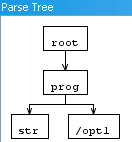input: str /opt1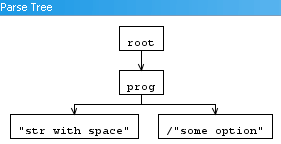"str with space" /"some option"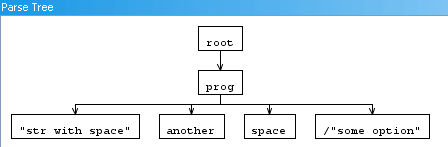"str with space" another space /"some option"

Conclusion:

No explicit need to include the WS in your lexer rule. “WS” will result in a token and splits input such as  “a b c d” (quote not include.

## Calculating / Computing the Bounding Box of Cubic Bezier

Goal: Showing an algo that computes the Bounding box of a cubic bezier.
Audience: Needs some basic Math, Derivative, solving parabola, knowledge of computer language.
Sample in c# : coming soon, at bottom of article.

This is also my first usage of a HTML5 element, so IE users sorry!

If you are NOT familiar with what a cubic bezier is, please look at this wiki page.

If you use the start and end point of a cubic bezier and both of the controlpoints, you will end up with a box. This box will contain the bezier, but it’s not really tight. You want a tight bb (bounding box). The controlpoints of the bezier may lay outside the bb.

This example is shows a working example (if the HTML5 canvas element is supported you’ll see a interactive demonstration) :

Sorry the CANVAS tag is not supported by your browser (are you browsing with IE??) 🙁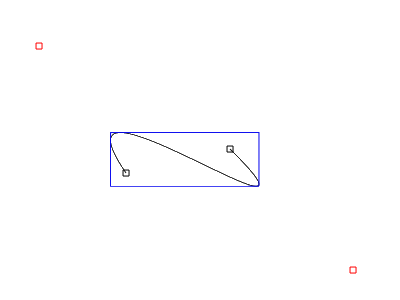So how to do this?

You’ll need to know the function of the cubic bezier. Defined as:
f(t) = a*t^3 + b*t^2 + c*t +d
where
d = P0
c = 3*P1-3*P0
b = 3*P2-6*P1+3*P0
a = P3-3*P2+3*P1-P0

p0 and p3 are the begin and end point of the bezier, p1 & p2 the control points. This function is defined for t=[0..1] so (0, 0.00001, 0.00002, … 0.99999)

Code to draw bezier point by point

```
protected override void OnPaint(PaintEventArgs pe)
{
Func<double, double, double, double, double, double> bezierSpline = (p0, p1, p2, p3, t) =>
(p3 - 3 * p2 + 3 * p1 - p0) * Math.Pow(t, 3)
+ (3 * p2 - 6 * p1 + 3 * p0) * Math.Pow(t, 2)
+ (3 * p1 - 3 * p0) * t
+ (p0);

for (double t = 0; t < = 1; t += 0.001) //adjust if you want
{
double x = bezierSpline(_points.X, _points.X, _points.X, _points.X, t);
double y = bezierSpline(_points.Y, _points.Y, _points.Y, _points.Y, t); ;

var p = new PointF((float)x, (float)y);
drawPoint(pe.Graphics, p, Color.Orange, 2);
}
}

private void drawPoint(Graphics g, PointF p, Color color, float size)
{
Brush b = new SolidBrush(color);
float w = size;
RectangleF r = new RectangleF(p.X-(w/2f),p.Y-(w/2f),w,w);
g.FillEllipse(b,r);
b.Dispose();
}//this code snippet has been cut-n-pasted from project, may contain some err..
```

A possible way to compute bb is to add each point to bb evaluation, and you need plenty to ensure the exactness of the bb! Don't do this! Unless you want to do TDD and test if the bb is nearly the same as the bb you are about to compute for testing purposes.

### Derivative

The exact solution can be found by using math. if you derivative the bezier function you can find local extremes.

so
f(t) = a*t3 + b*t2 + c*t +d
becomes :
f(t)' = 3a*t^2 + 2b*t + c

Do not forget the values of a,b and c! as defined above.

If you find the where f(t)'=0 then you'll find local extremes. To do this you'll need to find where the parabola equals 0 for each of the axises. You'll need to solve the parabola (f(t)' is now a parabola!) for the x and y values of your points.

To find out if there is a solution we'll use the discriminant (Quadratic formula) b^2-4ac. This will tell you if there is a solution.

Example:
suppose points:
p0 = (30,70)
p1 = (0,270)
p2 = (290,110)
p3 = (200, 100)

f(t)' = 3a*t^2 + 2b*t + c

with:

a = P3-3*P2+3*P1-P0
b = 3*P2-6*P1+3*P0
c = 3*P1-3*P0

becomes:

f(t)' = 3(P3-3*P2+3*P1-P0)*t^2 + 2(3*P2-6*P1+3*P0)*t + (3*P1-3*P0)

//solution for x values:
a = 3(200-3*290+3*0-30) = -2100
b = 2(3*P2-6*P1+3*P0) = 1920
c = (3*P1-3*P0) = -90

with D = b^2-4ac
D=2930400
D>0 so there are 2 solutions

you can calculate the solution with (-b + sqrt(b^2 - 4ac)) / 2a and (-b - sqrt(b^2 - 4ac)) / 2a.

s1 = 0.04956 s2 = 0.86472

do the same for the Y values
you'll come to:
a=1530
b=-2160
c=600

D=993600 so 2 solutions

s1=1.03163
s2=0.38013

!!You'll need to throw away the first solution of 1.03163, since the bezier function is only defined between 0 and 1!

us the solutions you came up as params for the original bezier function.
so :
//s1 = 0.04956 s2 = 0.86472
x1 = f(0.04956) => 27.8
y1 = f(0.04956) => 97.1

x2 = f(0.86472) => 217.3
y2 = f(0.86472) => 111.0

//s1=1.03163 s2=0.38013
x3 = f(0.38013) => 96.0
y3 = f(0.38013) => 170.0

ok,.. now you have the local extremes! And evaluation of the bb is next.

### The Bounding Box

Take the start and end point, include the local extremes.

find the smallest x and y value
find the largest x and y value.

Now there is a small trap,..
your p0..p3 can be placed in such a way that for the f(t)' a becomes 0. When this happens the function is no longer a parabola, but a line.
f(t)' = at^2 + bt + c
f(t)' = at^2 + bt + c
f(t)' = bt + c
-bt= c
-t = c/b
t = -c/b

that is all you'll need to solve the bb of a bezier!

## C# saving as EMF

This is a useful piece of code, created as an extension method on MetaFile to save a Metafile as a real Vector based emf not as a PNG. See : msdn on image encoders / decoders.

Here is the code:

```using System;
using System.Collections.Generic;
using System.Linq;
using System.Text;
using System.Runtime.InteropServices;
using System.IO;

namespace System.Drawing.Imaging
{
public static class ExtensionMethods
{
public static void SaveAsEmf(this Metafile me, string fileName)
{
How to save or serialize a Metafile: Solution found
by : SWAT Team member _1
Date : Friday, February 01, 2008 1:38 PM
*/
int enfMetafileHandle = me.GetHenhmetafile().ToInt32();
int bufferSize = GetEnhMetaFileBits(enfMetafileHandle, 0, null); // Get required buffer size.
byte[] buffer = new byte[bufferSize]; // Allocate sufficient buffer
if (GetEnhMetaFileBits(enfMetafileHandle, bufferSize, buffer) <= 0) // Get raw metafile data.
throw new SystemException("Fail");

FileStream ms = File.Open(fileName, FileMode.Create);
ms.Write(buffer, 0, bufferSize);
ms.Close();
ms.Dispose();
if (!DeleteEnhMetaFile(enfMetafileHandle)) //free handle
throw new SystemException("Fail Free");
}

[DllImport("gdi32")]
public static extern int GetEnhMetaFileBits(int hemf, int cbBuffer, byte[] lpbBuffer);

[DllImport("gdi32")]
public static extern bool DeleteEnhMetaFile(int hemfbitHandle);
}
}
```

How to use this code?

```            //Set up reference Graphic
Graphics refG = this.CreateGraphics(); //assumin this code is running on a control/form
IntPtr refGrap = refG.GetHdc();
var img = new Metafile(refGrap, EmfType.EmfPlusDual, "..");

//Draw some silly drawing
using (var g = Graphics.FromImage(img))
{
var r = new Rectangle(0,0,100,100);
var reye1 = new Rectangle(20, 20, 20, 30);
var reye2 = new Rectangle(70, 20, 20, 30);

var pBlack = new Pen(Color.Black, 3);
var pRed = new Pen(Color.Red, 2.5f);

g.FillEllipse(Brushes.Yellow, r);
g.FillEllipse(Brushes.White, reye1);
g.FillEllipse(Brushes.White, reye2);
g.DrawEllipse(pBlack, reye1);
g.DrawEllipse(pBlack, reye2);
g.DrawBezier(pRed, new Point(10, 50), new Point(10, 100), new Point(90, 100), new Point(90, 50));
}

refG.ReleaseHdc(refGrap); //cleanup
refG.Dispose();

img.SaveAsEmf("test.emf");  //chose this line

//img.Save("test2.emf", ImageFormat.Emf); //or this line
```

The end result:## Lexer At work, a tricky sample

I was asked to help out with a grammar. It’s part of the “Package Manager Specification” see gentoo’s spec.

The spec allow to add dependencies, the syntax for this is : [operator][category]/[package name]-[version]

 operator operator’s valid values are : ‘<‘ ‘<=’ ‘=’ ‘~’ ‘>=’ ‘>’ category 2.1.1 Category Names A category name may contain any of the characters [A-Za-z0-9+_.-]. It must not begin with a hyphen or a dot. Note: A hyphen is not required because of the virtual category. Usually, however, category names will contain a hyphen. package name 2.1.2 Package Names A package name may contain any of the characters [A-Za-z0-9+_-]. It must not begin with a hyphen, and must not end in a hyphen followed by one or more digits. version 2.2 Version Specifications The package manager must not impose fixed limits upon the number of version components. Package managers should indicate or reject any version that is invalid according to these rules. A version starts with the number part, which is in the form [0-9]+(n.[0-9]+)* (a positive integer, followed by zero or more dot-prefixed positive integers). This may optionally be followed by one of [a-z] (a lowercase letter). In the spec there are more requirements, but it will make this example so much more harder to understand.

now the trick is that package name (or pn for short), can not end in a hyphen followed by one or more digits’. Thus valids could be:

foo
foo-bla
foo1-bla-

but I’m not sure about the following.

foo-12a-12, if one follows the spec, it ends with -12.. so one could cut that of but you will still be left with -12a ,” a hyphen followed by one or more digits“. I would like to know it that is valid or not. Please tell me! I’m not sure.

I would like to know if this is valid: foo-123-foo? (does not end in.. I know)
I would like to know if this is valid:foo-12-12a

since category and pn and version are much alike and share much the same tokens it seemed logical to create parser rules instead of lexer rules.

dep    :    OPERATOR catagory SLASH pn HYPHEN version;

where OPERATOR is defined as:

OPERATOR:    ‘<‘|'<=’|’=’|’~’|’>=’|’>’;

and SLASH and HYPHEN :

HYPHEN    :    ‘-‘;
SLASH    :    ‘/’;

now here comes the difficult stuff:

Both Category and Pn share a lot of the same tokens. Category may contain a ‘.’  and pn may not. So if we would lex :
‘foo-bar’
would that result in  a CATEGORY token or a PN token? The answer is that Antlr would not stand by this and tell you that “the following token definitions can never be matched because prior tokens match the same input”. If there is no dot in the characters being lexed it could well have been a pn. In short both rules are quite ambigous, but it get’s wores. How do we tell the lexer the differences between a version and the pn if only characters are used that are valid for both rules. The answer is we need to do this ourselfs. The lexer will happely continue eating valid chars and thus eating characters in the version portion. It would have been easier if the final hypen would have been a character not in the range of valid chars of eiter rule.

On the other hand CATEGORY always comes after OPERATOR and PN always after SLASH. We can uses this to our advantage by using a construct named ‘semantic predicates’. We are going to use this to influence the lexer.

When the lexer is done wit operator it will set a flag telling it to match the tokens as with the category rules, and after the slash the flag will be set again to match tokes that apply to the package name rules. Resulting in “OPERATOR:    (‘<‘|'<=’|’=’|’~’|’>=’|’>’ ){category=true;};” and “SLASH    :    ‘/’  {category=false;};”.

a boolean will be set to true or to false. Which has still to be defined at the lexer by setting (basic programming rule:”declare what you want to use”) :

@lexer::members
{
boolean category=false;
}

Now Category and pn share common tokens, these are defined as follows:
fragment GENERIC    :    (UPPER|LOWER|UNDERSCORE|DIGIT);

(i use fragment so GENERIC won’t be seen as a individual token, it can be used over and over again.)

Next we are putting both

STR:
GENERIC
(
{category}?=>(GENERIC|PLUS|DOT|HYPHEN)*
|
{!category}?=>(GENERIC|PLUS|HYPHEN)*
)

So if we now set dep to : “dep    :    OPERATOR category SLASH pn; // HYPHEN VERSION;” (skipping the version) and test it: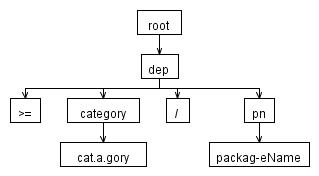Input was “>=cat.a.gory/packag-eName” Input was fully taken correctly.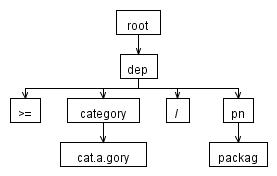Input was “>=cat.a.gory/packag.eName” The lexer stopped at “packag” since ‘.’ was not a valid token for package name.

Now we can add version to it, thus changing dep to : “dep    :    OPERATOR category SLASH pn HYPHEN VERSION;” ,  and try “>=cat.a.gory/packageName-12a” for inpu, telling it’s version 12.Whoa what just happend?!! package name included the version!

The cause to this is because the “-12a” part can be consumed by the package name rule. And the “can not end with hyphen followed by digit” rule was not implemented.

How to implement can not end? Well currently Antlr does not support such a construct as in may not end in (not to be confused by the ‘~’ operator, meaning match anything until …). I’m going to use the input.mark, input.rewind and input release commands.  When mark is called, you get an id. With this id you can rewind back to this mark. Any tokens matched in between will become consumable once again, it’s like reversing time. Input.Release(id) does not rewind, but cleans up the administration.

The goal is to match “packageName-12a”, with a marker @ the HYPEN. Then we check if the rule “can not end in hypen.. digit” is true or false. If true, we will simply rewind and the that’s that.  So the matched “packageName-12a” will end up in “packageName” (with “-12a” rewinded, left for other rules).

Every time a hyphen comes by i’ll mark it, if we already had a mark, release it.

This is the final result:

//options
//{
//language = CSharp;
//}

//http://www.gentoo.org/proj/en/qa/pms.xml

/*
2.1.1 Category Names
A category name may contain any of the characters [A-Za-z0-9+_.-]. It must not begin with a hyphen or a
dot.
Note: A hyphen is not required because of the virtual category. Usually, however, category names will
contain a hyphen.
*/

/*
2.1.2 Package Names
A package name may contain any of the characters [A-Za-z0-9+_-]. It must not begin with a hyphen, and
must not end in a hyphen followed by one or more digits.
*/

/*
2.2 Version Specifications
The package manager must not impose fixed limits upon the number of version components. Package managers
should indicate or reject any version that is invalid according to these rules.
A version starts with the number part, which is in the form [0-9]+(n.[0-9]+)* (a positive integer, followed
by zero or more dot-prefixed positive integers).
This may optionally be followed by one of [a-z] (a lowercase letter).
*/

@lexer::members
{
boolean category=false;

//c#
//bool category=false;

}

dep    :    OPERATOR category SLASH pn HYPHEN VERSION;

HYPHEN    :    ‘-‘;
SLASH    :    ‘/’  {category=false;};
VERSION    :    DIGIT+ (DOT DIGIT+)* LOWER?;
OPERATOR:    (‘<‘|'<=’|’=’|’~’|’>=’|’>’) {category=true;};

category:    STR;
pn    :    STR;

STR
@init
{
int _mrk=-1;
//    int start = CharIndex; //c#
int start = getCharIndex(); //JAVA
}
:
//c#    {int _mrk=-1;int start = getCharIndex;}

GENERIC
(
{category}?=>(GENERIC|PLUS|DOT|HYPHEN)*
|
{!category}?=>
(

(GENERIC|PLUS|( {if (_mrk!=-1){input.release(_mrk);} {_mrk = input.mark();} }  HYPHEN))*
)
)
/*
Post Check: If this is a Package name and it ends in eg. -12 just take that off.
*/
{
if (!category)
{

String s = input.substring(start, input.getCharPositionInLine()-1);
String[] s2 = s.split(“-“);
if ( Character.isDigit(s2[s2.length-1].charAt(0)) && s2.length>1)
{
input.rewind(_mrk); _mrk = -1; //rewind (altering this tokens, leave the rest to be matched.)
}
else
{
if (_mrk != -1) { input.release(_mrk); } //release, do not alter token.
}

}
}//end of post check.
;

fragment UNDERSCORE    :    ‘_’;
fragment UPPER    :    ‘A’..’Z’;
fragment DIGIT    :    ‘0’..’9′;
fragment LOWER     :    ‘a’..’z’;
fragment DOT    :    ‘.’;
fragment PLUS    :    ‘+’;
fragment GENERIC    :    (UPPER|LOWER|UNDERSCORE|DIGIT);

WS     : (‘ ‘|’t’ | ‘r’ | ‘n’)+ {\$channel=HIDDEN;};

LICENSE: All source code in this post comes with ABSOLUTELY NO WARRANTY, This is free software, and you are welcome to redistribute it under certain conditions, see GPL 2.

## DrawCurve / AddCurve for WPF / Cardinal Spline

For my project I need to draw Cardinal splines. Preferable the same way I was used to with the System.Drawing.Graphics or System.Drawing.Drawing2D.GraphicsPath. Not knowing much about splines, I noticed the number of PathPoints on a graphics path. The curve is drawn with beziers, so it’s some sort of string of beziers that make up this cardinal spline.

This project results in the same curves as you are used to with the graphics object (but this works with doubles not with floats). Tension and open and closed params are supported!

Anyway long story short, this project calculates control points for beziers. The curve will be draw with beziers, thus the outcome is exactly the same as with the Graphics object. I’ve seen other examples drawing the curve by them self calculating 100’s of points on the bezier it self .

Yes the graphics object does the same thing see msdn (quote:”Remarks The user must keep the original points if they are needed. The original points are converted to cubic Bézier control points internally, therefore there is no mechanism for returning the original points.”) .

If you draw a curve with 3 points, 7 points are needed to draw the curve, thus a performance gain(? not measured!) without losing the nice smoothness of the curve.

here is a screen shot: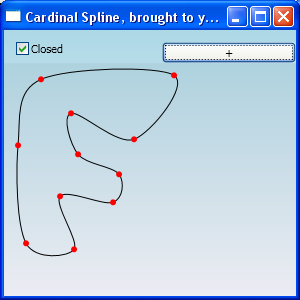comments are welcome, Can u use this, or are you missing something?

Tags
Categories
Meta